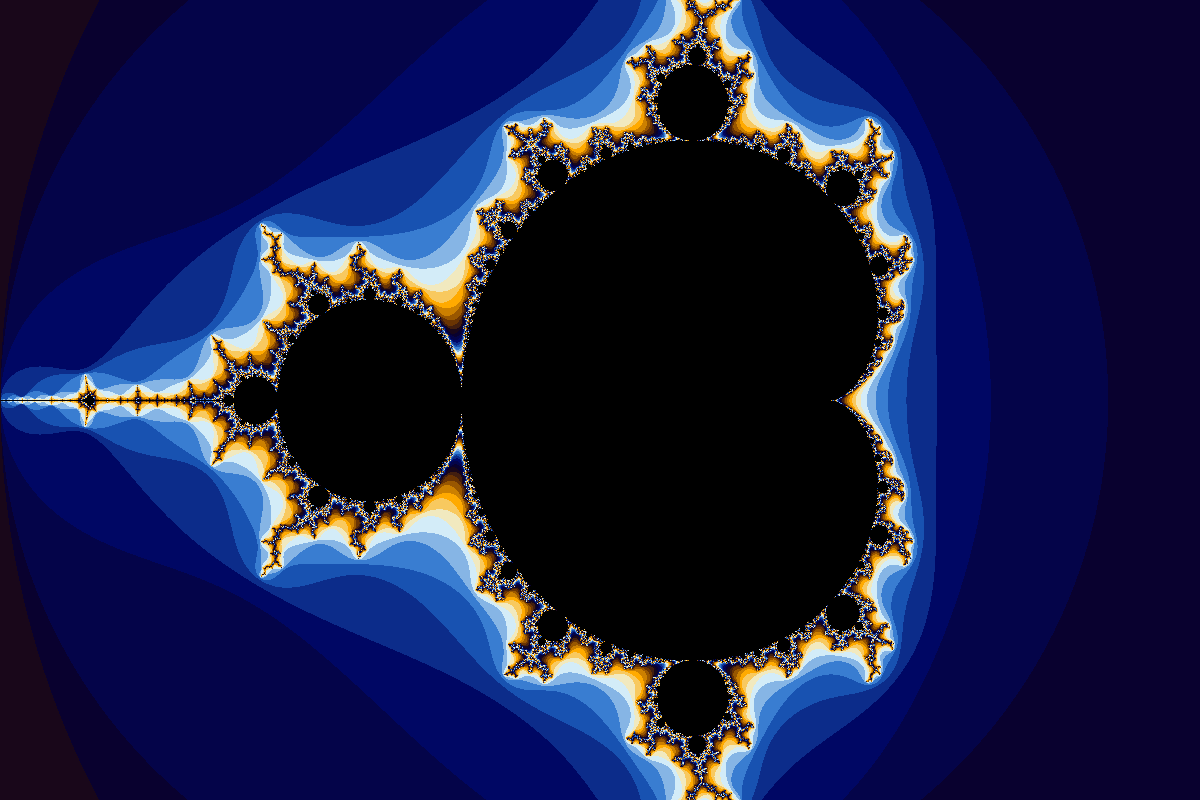This weekend I decided to play around with OpenCL, something I had never used before. As first project I built a Mandelbrot set renderer. This seemed fitting, since in high school I wrote an extremely slow version in TI Basic on a TI84 graphic calculator. Rendering a single image took about 45 minutes.

The math behind the Mandelbrot set is relatively simple. A point $$c$$ on the complex plane is included in the set if the sequence $$z_{n+1} = z_n^2 + c$$ with $$z_0 = 0$$ is bounded. For simplicity we interpret “bounded” as $$\vert z\vert < 4$$ after 256 iterations. To add some nice coloring to the points outside of the set, we can count how many iterations it takes for the sequence to go “unbounded”.

I translated the pseudo code from Wikipedia into the following OpenCL kernel:

__kernel void mandelbrot(__write_only image2d_t out) {
int2 pos = (int2)(get_global_id(0), get_global_id(1));
int2 size = get_image_dim(out);

// Scale x to -2.5 to 1
float x0 = (float)pos.x / size.x;
x0 = x0*3.25 - 2;

// Scale y to -1 to 1
float y0 = (float)pos.y / size.y;
y0 = y0*2.0 - 1.0;

float x = 0.0;
float y = 0.0;

uint max_its = 256;
uint i = 0;
float d = 0.0;

while (i < max_its && d < 4.0){
float xtemp = x*x - y*y + x0;
y = 2*x*y + y0;
x = xtemp;

d = x*x + y*y;
i++;
}

uint4 color = (255, 255, 255, 255);

if (d < 4.0){
color.xyz = (uint3)(0, 0, 0);
} else {
color.xyz = get_color(i);

}

write_imageui(out, pos, color);
}

The complete code can be found on my GitHub repo.